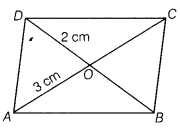# Diagonals AC and BD of a parallelogram ABCD intersect each other at O

Diagonals AC and BD of a parallelogram ABCD intersect each other at O. If OA=3 cm and OD = 2 cm, determine the lengths of AC and BD.

Given, OA = 3 cm and OD =2 cmWe know that diagonals of a parallelogram bisect each other.
AC = 2 OA = 6 cm
and BD =2 OD = 4 cm